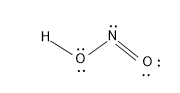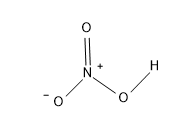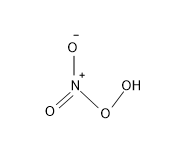Name three Oxo acids of nitrogen.Verified
119.1k+ views
Hint:. Oxy acids or Oxo acids are the acids that contain oxygen atoms in them.
They will have a general formulae of X-O-H

Oxyacids are ternary acids with oxygen atoms. For every Oxo acid the O and H atoms is a prerequisite condition. The H and O atoms present are connected to a central atom, say X.
So the general formulae for the Oxo acid is X-O-H.
The Oxo acids in the solution dissociates in two ways,
$X-O-H\rightleftarrows {{(X-O)}^{-}}+{{H}^{+}}$
$X-O-H\rightleftarrows {{X}^{-}}+O{{H}^{-}}$
And the dissociation depends on the central atom If the central atom is more electronegative atom than O then the dissociation will be like that of an acid i.e. it produces ${{H}^{+}}$ ions. If the X atom is less electronegative than O then it will be similar to base.
Oxo acids of Nitrogen are those Oxo acids having the central metal atom as N in them.
Some of the very common oxoacids of Nitrogen are –
-Nitrous acid
-Nitric acid
-Hyponitric acid
-Peroxy nitric acid
-Peroxynitrous acid etc.
Nitrous acid -$HN{{O}_{2}}$
The nitrous acid in gaseous is prepared by the following reaction,
$NO+N{{O}_{2}}+{{H}_{2}}O\rightleftarrows 2HN{{O}_{2}}$
It is a monoprotic weak acid and it yields nitrite ion$\left( N{{O}_{2}}^{-} \right)$ by ionization.
- Nitrous acid is used for the preparation of diazonium salts from amines, which in turn is used for the coupling reaction of azo compounds as reagents for the preparation of azo dyes.
The structure of nitrous acid is as follows:Nitric acid - $HN{{O}_{3}}$
- Nitric acid is one of the most familiar strong acids. It is also called aqua Fortis which means strong water in Latin.
- It is colorless in its pure form, with the course of time the colour changes to yellow. $HN{{O}_{3}}$ is prone to thermal decomposition into oxides of N and to water, hence they are stored in dark colored bottles.
- $HN{{O}_{3}}$ is used for the nitration process and it is a very good oxidizing agent.
- $HN{{O}_{3}}$ is prepared by dissolving $N{{O}_{2}}$ in water.
The reaction is, $3N{{O}_{2}}+{{H}_{2}}O\to HN{{O}_{3}}+NO$
The structure of $HN{{O}_{3}}$ is:Peroxynitric acid - $HN{{O}_{4}}$
- Pernitric acid or peroxy nitric as is one of the oxoacids of N.
- It is a very explosive acid, even though it is unstable it is a good source of $N{{O}_{2}}$, through a reverse radical mechanism happening in the atmosphere.
$H{{O}_{2}}N{{O}_{2}}\rightleftarrows HO_{2}^{\bullet }+NO_{2}^{\bullet }$
The structure of $HN{{O}_{4}}$ is:Note: The oxidation of N in $HN{{O}_{2}}$ is +3, in $HN{{O}_{3}}$ is +5 and in $HN{{O}_{4}}$ is +1.